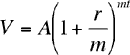Quandaries and Queries Dear Sir or Madam: I recently received \$7,987.68. This amount consisted of \$7,543.61 invested at 6% per annum, compounded daily. I am trying to find out how many days this investment accrued interest. Thank you, Bruce Hi Bruce, If you invest \$A at an annual interest rate of r per anum, compounded m times a year then the value after t years is \$V given byYou have \$V = \$7987.68, \$A = \$7543.61, r = 0.06 and m = 365. I substituted the values, divided both sides by A and then took the log of both sides to get 0.02484143 = mt (0.000071385) and hence mt = 347.99 days Cheers,Penny Go to Math Central